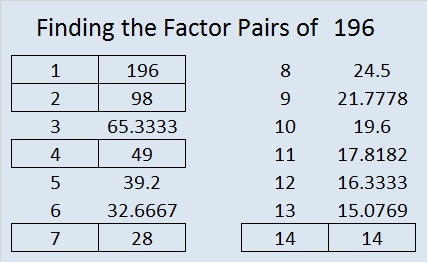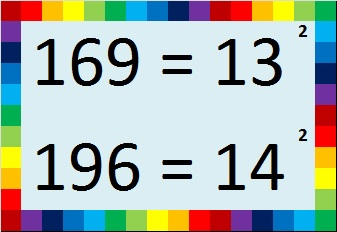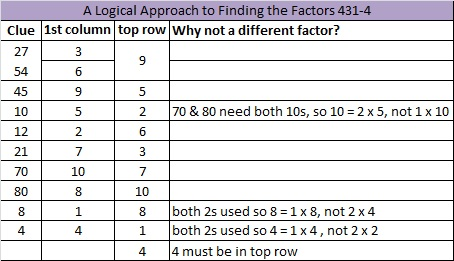# 196 and Level 4

• 196 is a composite number.
• Prime factorization: 196 = 2 × 2 × 7 × 7, which can be written 196 = 2² × 7²
• The exponents in the prime factorization are 2 and 2. Adding one to each and multiplying we get (2 + 1)(2 + 1) = 3 × 3 = 9. Therefore 196 has exactly 9 factors.
• Factors of 196: 1, 2, 4, 7, 14, 28, 49, 98, 196
• Factor pairs: 196 =  1 × 196, 2 × 98, 4 × 49, 7 × 28, or 14 × 14
• 196 is a perfect square. √196 = 14Excel file of puzzles and previous week’s factor solutions: 10 Factors 2014-08-04